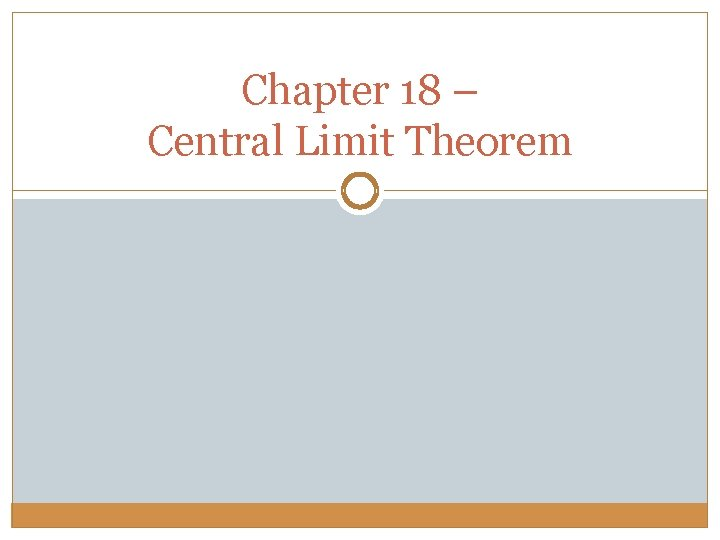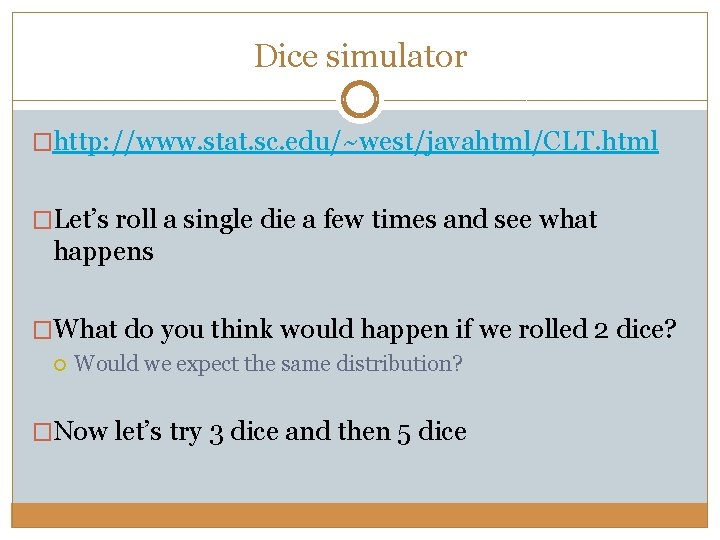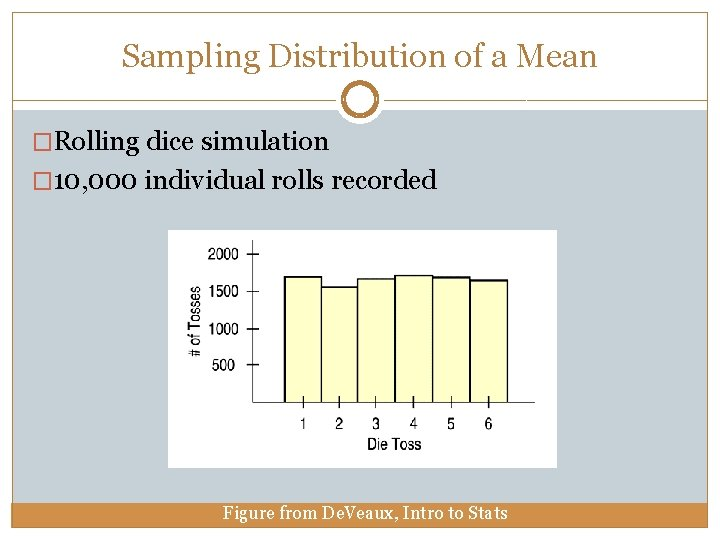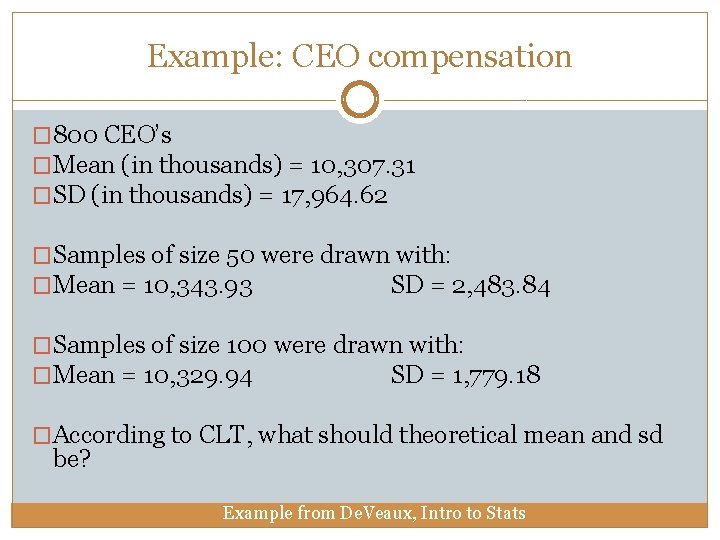# Chapter 18 Central Limit Theorem Dice simulator http

• Slides: 13Chapter 18 – Central Limit TheoremDice simulator �http: //www. stat. sc. edu/~west/javahtml/CLT. html �Let’s roll a single die a few times and see what happens �What do you think would happen if we rolled 2 dice? Would we expect the same distribution? �Now let’s try 3 dice and then 5 diceNFL Data �Minitab data setSampling Distribution of a Mean �Rolling dice simulation � 10, 000 individual rolls recorded Figure from De. Veaux, Intro to StatsSampling Distribution of a Mean �Roll 2 dice 10, 000 times, average the 2 dice Figure from De. Veaux, Intro to StatsSampling Distribution of a Mean �Rolling 3 dice 10, 000 times and averaging the 3 dice Figure from De. Veaux, Intro to StatsSampling Distribution of a Mean �Rolling 5 dice 10, 000 times and averaging Figure from De. Veaux, Intro to StatsSampling Distribution of a Mean �Rolling 20 dice 10, 000 times and averaging �Once again, as sample size increases, Normal model appears Figure from De. Veaux, Intro to StatsCentral Limit Theorem �The sampling distribution of any mean becomes more nearly Normal as the sample size grows. The larger the sample, the better the approximation will be Doesn’t matter what the shape of the distribution of the population is! (uniform, symmetric, skewed…) �Observations need to be independent and collected with randomization.CLT Assumptions �Assumptions: Independence: sampled values must be independent Sample Size: sample size must be large enough �Conditions: Randomization 10% Condition Large enough sampleWhich Normal Model to use? �The Normal Model depends on a mean and sd �Sampling Distribution Model for a Mean When a random sample is drawn from any population with mean µ and standard deviation σ, its sample mean y has a sampling distribution with: Mean: µ Standard Deviation:Example: CEO compensation � 800 CEO’s �Mean (in thousands) = 10, 307. 31 �SD (in thousands) = 17, 964. 62 �Samples of size 50 were drawn with: �Mean = 10, 343. 93 SD = 2, 483. 84 �Samples of size 100 were drawn with: �Mean = 10, 329. 94 SD = 1, 779. 18 �According to CLT, what should theoretical mean and sd be? Example from De. Veaux, Intro to StatsNormal Models �Binomial µ = np σ= �Sampling distribution for proportions �Sampling distribution for means SD(y) =